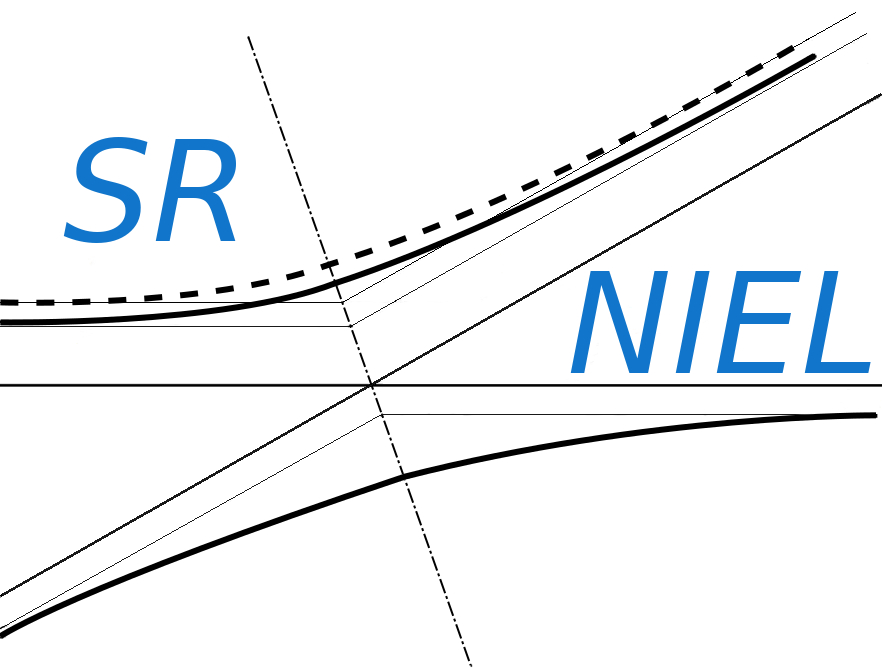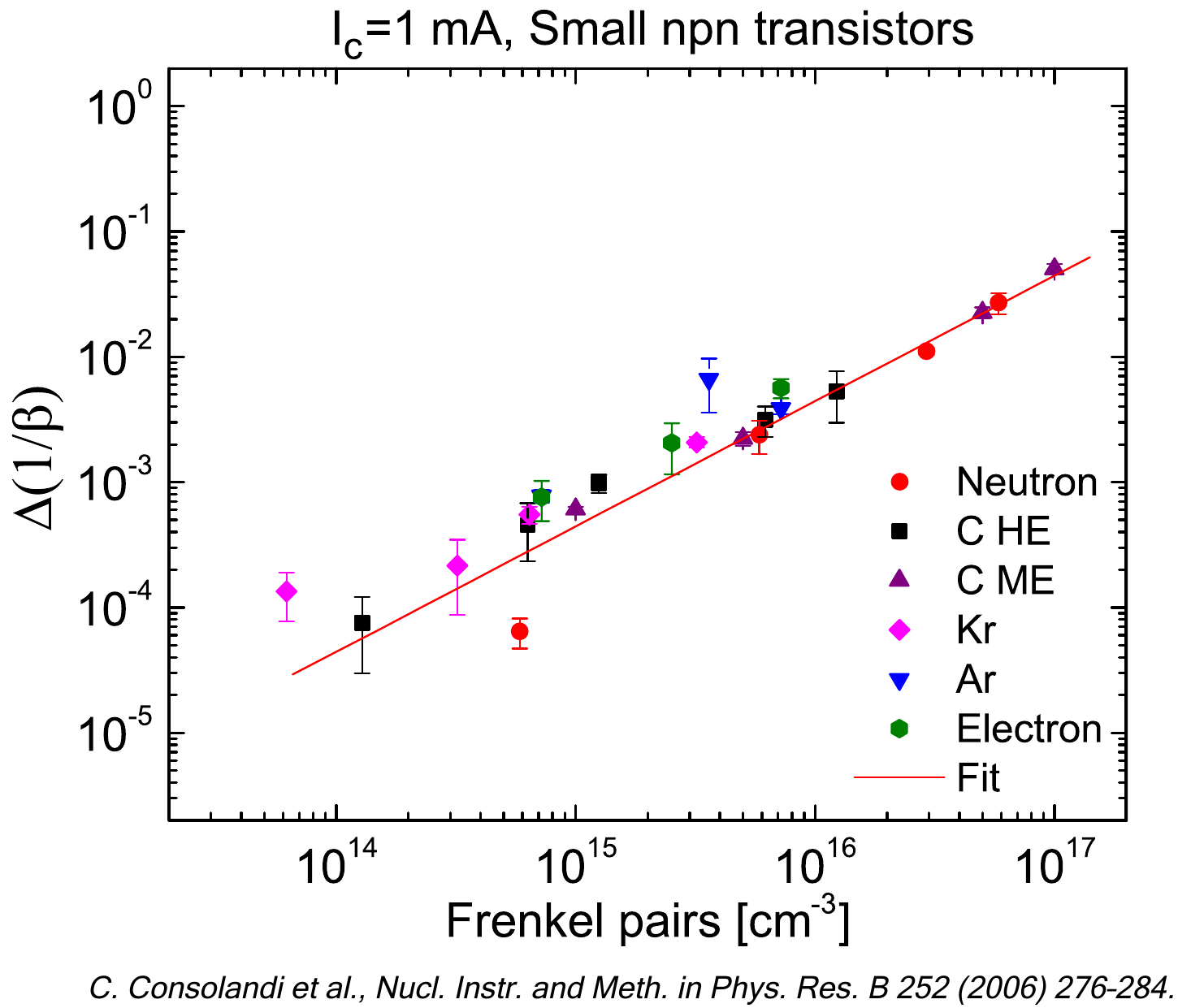SR-NIEL – 7 Screened Relativistic (SR) Treatment for NIEL Dose Nuclear and Electronic Stopping Power Calculator (version 8.0)The present Calculator provides the "total probability in g-1" of energetic recoil nuclei due to a spectral fluence.

### How to use this Recoil Probability Calculator

The Probability (per cm2) for an incoming fluence of particle to generate recoil nuclei due to Coulomb interaction above the requested Eener value is derived by:

(total probability in g-1) x (absorber density in g cm-3) x (traversed thickness in cm),

where the "total probability in g-1" is obtained by the web calculator, absorber density and traversed thickness are defined by the user.
Eener is the the lower limit of recoil energy. For information about the computation method one may see the Energetic Nuclear Recoil page.

The recoil energy is deposited by ionizing and non-ionizing processes. For every recoil energy value, the amounts of energy deposited for those processes  are available, for elements, at Energy Partition Function Calculator.

Input parameters and options for the tool are described below. When the input form has been completed, pressing the "CALCULATE" button will start the calculation and open the "Results" page (allow for pop-up in your browser settings). The result page will be also linked at the bottom of the calculator page.

For further information about the computation method are available at the Nuclear Stopping Power for Electrons, Protons and Ions page.

### Input Parameters:

- Incident particle (for Protons&Ions Calculator)
- Electron form (for Electrons Calculator)
- Target material

### Incident Particle

In the Protons&Ions Calculator, using the pull down menu, the user can select the species of the incident particle, either a proton or one of the elemental ions.

### Electron Form Factor

In the Electron Calculator, the user can select the nuclear form factor (Exponential, Gaussian or Uniform ) model to be used. The form factor accounts for the spatial distribution of charge density probed in the electron-nucleus scattering.

### Target Material

In this section it is possible to specify the composition of the target material selecting the number of elements in the compound. The required parameters for each element are:

- Atomic number (Z)/Chemical symbol
- Stoichiometric index or element fraction
- Lower limit of the recoil energy Eener

### Particle Spectral Fluence

This section define the points of the spectral fluence as a function of energy.
The input format is one point per line (Energy - Spectral Fluence , separated by a space or tab); it is also possible to copy and paste values.

### Result

The result page contains the input parameters, the input spectral fluence and the recoil "probability in cm2 g-1" (i.e., that for a single particle)  calculated at the same energies defined in the spectral fluence. For every energy points the spectral probabilty is calculated and displayed in the second graph. The spectral probability is integrated, at the energy steps defined in the spectral fluence, to give "total probability in g-1". The result tables include the spectral fluence, recoil probabilty and spectral total probability.